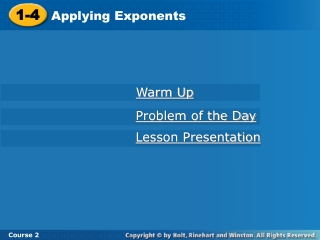# 1-4 - PowerPoint PPT PresentationDownload Presentation1-4

1-4
Download Presentation## 1-4

- - - - - - - - - - - - - - - - - - - - - - - - - - - E N D - - - - - - - - - - - - - - - - - - - - - - - - - - -
##### Presentation Transcript

1. 1-4 Applying Exponents Course 2 Warm Up Problem of the Day Lesson Presentation

2. Warm Up Find each value. 1.92 3. 152 5. 103 81 2. 122 144 225 4. 102 100 1,000 10,000 6. 104

3. Problem of the Day Each day, Lowell runs one more lap than he did the day before. After seven days he has run a total of 77 laps. How many laps did he run on the first day? 8

4. Learn to express large numbers in scientific notation.

5. Vocabulary scientific notation

6. The distance from Venus to the Sun is over 100,000,000 kilometers. You can write this number as a power of ten by using a base of ten and an exponent. 10 · 10 · 10 · 10 · 10 · 10 · 10 · 10 = 108 Power of ten

7. The table shows several powers of ten. Power of 10 Meaning Value 101 10 10 102 10 · 10 100 10 · 10 · 10 1,000 103 104 10 · 10 · 10 · 10 10,000

8. Additional Example 1A: Multiplying by Powers of Ten Multiply 14 · 103. Method 1: Evaluate the power. Multiply 10 by itself 3 times. 14 · 103 = 14 · (10 · 10 · 10) Multiply. = 14 · 1,000 = 14,000

9. Additional Example 1B: Multiplying by Powers of Ten Multiply 14 · 103. Method 2: Use mental math. Move the decimal point 3 places. (You will need to add 3 zeros.) 14 · 103 = 14.000 3 places = 14,000

10. Check It Out: Example 1A Multiply 12 · 102. Method 1: Evaluate the power. Multiply 10 by itself 2 times. 12 · 102 = 12 · (10 · 10) Multiply. = 12 · 100 = 1,200

11. Check It Out: Example 1B Multiply 12 · 102. Method 2: Use mental math. Move the decimal point 2 places. (You will need to add 2 zeros.) 12 · 102 = 12.00 2 places = 1,200

12. Scientific notation is a kind of shorthand that can be used to write large numbers. Numbers expressed in scientific notation are written as the product of two factors. In scientific notation, 17,900,000 is written as 7 1.79 x 10 A power of 10 A number greater than or equal to 1 but less than 10

13. Writing Math In scientific notation, it is customary to use a multiplication cross () instead of a dot.

14. 6 places Additional Example 2: Writing Numbers in Scientific Notation Write the number 4,340,000 in scientific notation. Move the decimal point to get a number that is greater than or equal to 1 and less than 10. 4,340,000 = 4,340,000 The exponent is equal to the number of places the decimal point is moved. = 4.34  106

15. 6 places Check It Out: Example 2 Write the number 8,421,000 in scientific notation. Move the decimal point to get a number that is greater than or equal to 1 and less than 10. 8,421,000 = 8,421,000 The exponent is equal to the number of places the decimal point is moved. = 8.421  106

16. Additional Example 3: Writing Numbers in Standard Form The population of China in the year 2000 was estimated to be about 1.262  109. Write this number in standard form. Since the exponent is 9, move the decimal point 9 places to the right. 1.262 109 = 1.262000000 = 1,262,000,000 The population of China was about 1,262,000,000 people.

17. Check It Out: Example 3 The distance from the Earth to the Sun is calculated to be 1.5  108 kilometers. Write this distance in standard form. Since the exponent is 8, move the decimal point 8 places to the right. 1.5 108 = 1.50000000 = 150,000,000 The distance from the Earth to the Sun is about 150,000,000 kilometers.

18. Mexico: 1.06  108 Brazil: 1.86  108 Notice that 1.06 < 1.86. So 1.06  108 < 1.86  108 Additional Example 4: Comparing Numbers in Scientific Notation In 2005, the population of Mexico was 1.06  108 and the population of Brazil was 1.86  108. In which country do more people live? To compare numbers written in scientific notation, first compare the exponents. If the exponents are equal, then compare the decimal portion of the numbers. Brazil has more people living there.

19. Ty’s jar: 0.76  104 Laurel’s jar: .93  103 Notice that 4 > 3. So .76  104 > .93  103 Check It Out: Additional Example 4 The number of coins in Ty’s jar was 0.76  104 and number of coins in Laurel’s jar was 0.93  103. In which jar are there more coins? To compare numbers written in scientific notation, first compare the exponents. If the exponents are equal, then compare the decimal portion of the numbers. Ty’s jar has more coins in it.

20. Lesson Quiz: Part I Multiply. 2,500 1. 25  102 2. 18  104 180,000 3. 110  102 11,000 4. 3.742  103 3,742

21. Lesson Quiz: Part II Write each number in scientific notation. 5. 7,400,000 6. 45,000 7. Earth is about 9.292  107 miles from the Sun. Write this number in standard form. 7.4  106 4.5  104 92,920,000